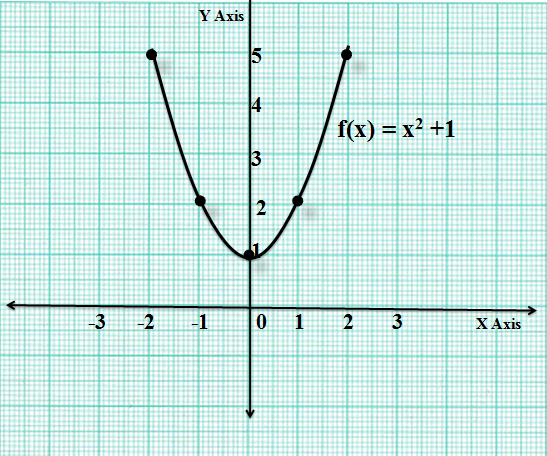## Algebra

#### FUNCTIONS-1

The general form of  a quadratic function is f(x) = ax2 + bx + c, where a, b and c are real numbers and a ≠ 0. The degree of the expression is two. i.e. the highest exponent of the variable is two. Here a, b are the coefficients of x2 , x and c is the constant term.

Let us say f(x) =  x2 + 1. Now if we plot the curve of f(x), we get a parabola:

 Input =  x -2 -1 0 1 2 Output = f(x) 5 2 1 2 5Here the vertex of the parabola lies at the point (0,1). The point of minima is x = 0 and the minimum value of f(x) = 1.

If f(x) = ax2 + bx + c = 0 , then it is called a quadratic equation. This means that the value of the quadratic expression is zero. Here we find the values of x for which the quadratic assumes a value equal to zero. In other words we can say that the values of x for which the graph of f(x) cuts the  X axis.